# 一种基于短路电抗器的直流配网故障保护方法研究Study on Fault Protection of DC Distribution System Based on Short-Circuit Reactor

• 全文下载: PDF(3205KB)    PP.516-524   DOI: 10.12677/SG.2018.86056
• 下载量: 370  浏览量: 562   科研立项经费支持

This paper analyzes the fault process of a multi-terminal DC power distribution system to obtain the equivalent circuit when the multi-terminal DC power distribution system fails, and then calculates the fault current of the multi-terminal DC power distribution system and analyzes the fault characteristics of the system. Through simulation, it is found that when the system fails, it is difficult to distinguish between bus faults and feeder faults based on the short-circuit current charac-teristics. Therefore, this article proposes to install a series reactor at the outlet of the feeder so that the short-circuit current of the bus fault and the short-circuit current of the feeder fault can be distinguished well in the amplitude characteristics and waveform characteristics, thus providing a criterion for the protection of the multi-terminal DC power distribution system.

1. 引言

2. 网架结构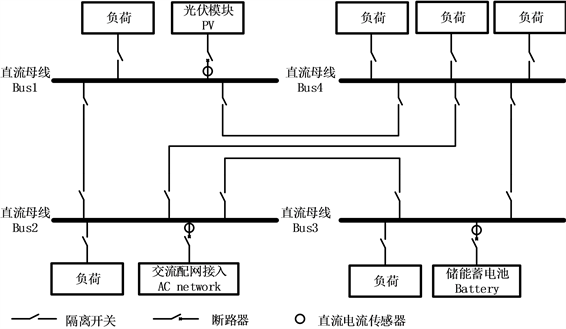Figure 1. Structure of multi-terminal DC distribution system

3. 等效电路与短路电流分析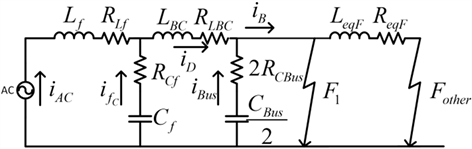Figure 2. Equivalent circuit of short-circuit fault on the side of AC distribution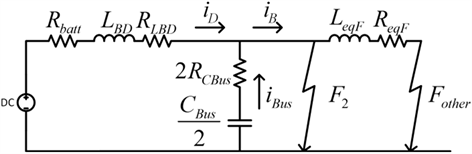Figure 3. Equivalent circuit of short-circuit fault on the side of energy storage

$\left\{\begin{array}{l}{i}_{D}={i}_{B}\\ {E}_{DC}-\left({R}_{batt}+{R}_{LBD}\right){i}_{D}-{L}_{BD}\frac{\text{d}i}{\text{d}t}=0\end{array}$ (1)

$\left\{\begin{array}{l}{i}_{D}={i}_{B}=\frac{{E}_{DC}}{{R}_{batt}+{R}_{LBD}}\left(1-{\text{e}}^{t/{\tau }_{D}}\right)\\ {\tau }_{D}=\frac{{L}_{BD}}{{R}_{batt}+{R}_{LBD}}\end{array}$ (2)

$\left\{\begin{array}{l}{i}_{D}={i}_{B}+{i}_{Bus}\\ {E}_{DC}-\left({R}_{batt}+{R}_{LBD}\right){i}_{D}-{L}_{BD}\frac{\text{d}{i}_{D}}{\text{d}t}-{U}_{d}=0\\ {U}_{d}-{R}_{eqF}{i}_{B}-{L}_{eqF}\frac{\text{d}{i}_{B}}{\text{d}t}-2{R}_{Bus}{i}_{Bus}-{U}_{{C}_{Bus}}=0\\ {i}_{Bus}=\frac{{C}_{Bus}}{2}\frac{\text{d}{U}_{{C}_{Bus}}}{\text{d}t}\end{array}$ (3)

$\left\{\begin{array}{l}{i}_{D}={D}_{1}{\text{e}}^{-{\tau }_{D}t}+{D}_{2}{\text{e}}^{-{\tau }_{D}t}\\ {D}_{1}={i}_{D0}\\ {D}_{2}={i}_{D0}{\tau }_{D}+\frac{{E}_{DC}-\left(2{R}_{Bus}+{R}_{eqF}\right)}{{L}_{eqF}}\\ {\tau }_{D}=\frac{2{R}_{Bus}+{R}_{eqF}}{2{L}_{eqF}}\end{array}$ (4)

4. 改进后的故障特征分析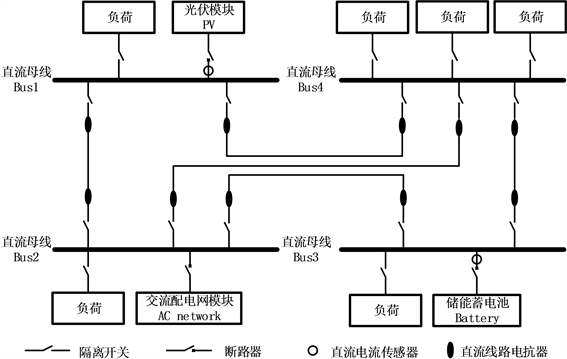Figure 4. Structure of multi-terminal DC distribution system with short-circuit reactor installedFigure 5. Equivalent circuit of short-circuit fault response on the side of AC distribution with short-circuit reactor installed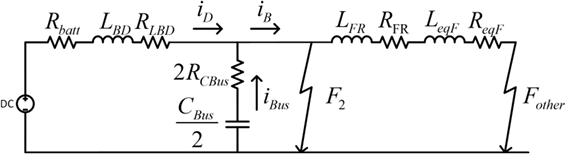Figure 6. Equivalent circuit of short-circuit fault response on the side of energy storage with short-circuit reactor installed

5. 仿真算例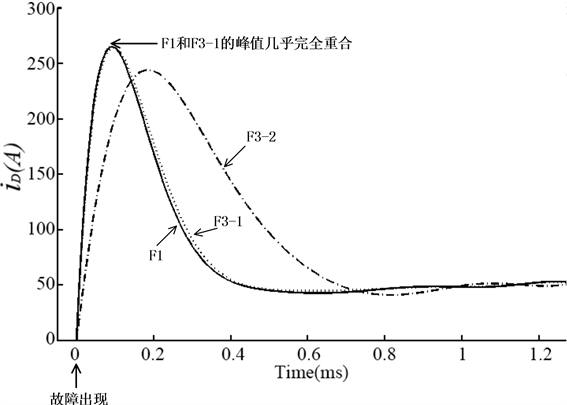Figure 7. Short-circuit current curve without short-circuit reactor installed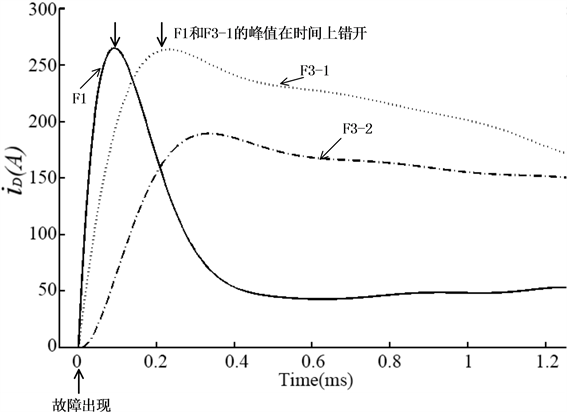Figure 8. Short-circuit current curve with different short-circuit reactor installedTable 1. Comparison of short-circuit peak value and differential quantity with short-circuit reactor installed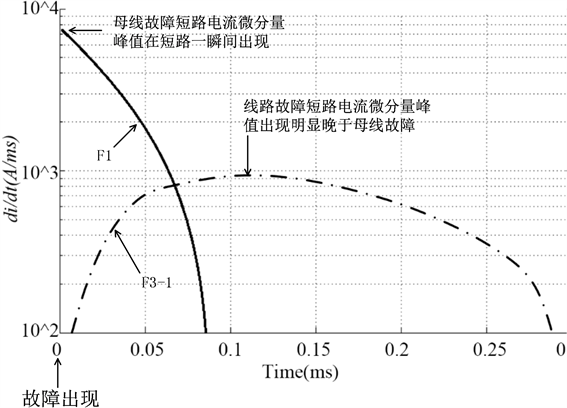Figure 9. Short-circuit current differential quantity curve with short-circuit reactor installed

6. 结论

  马钊, 焦在滨, 李蕊. 直流配电网络架构与关键技术[J]. 电网技术, 2017, 41(10): 3348-3357.  盛万兴, 李蕊, 李跃, 殷正刚, 吴鸣,孙丽敬, 张海. 直流配电电压等级序列与典型网络架构初探[J]. 中国电机工程学报, 2016, 36(13): 3391-3403, 3358.  Farhadi, M., Mohamed, A. and Mohammed, O. (2013) Connectivity and Bidirectional Energy Transfer in DC Microgrid Featuring Different Voltage Characteristics. Proceedings of the IEEE Green Technologies Conference, Denver, 244-249. https://doi.org/10.1109/GreenTech.2013.45  Salomonsson, D., Soder, L. and Sannino, A. (2009) Protection of Low-Voltage DC Microgrids. IEEE Transactions on Power Delivery, 24, 1045-1053. https://doi.org/10.1109/TPWRD.2009.2016622  朱建萍, 周建萍, 时珊珊, 等. 光伏发电接入直流配电网DC-DC变换器的仿真研究[J]. 广东电力, 2015, 30(2): 20-24, 33.  张文亮, 汤涌, 曾南超. 多端高压直流输电技术及应用前景[J]. 电网技术, 2010, 34(9): 1-6.  胡子珩, 马骏超, 曾嘉思, 等. 柔性直流配电网在深圳电网的应用研究[J]. 南方电网技术, 2014, 8(6): 44-47.  徐通, 王育飞, 张宇, 等. 直流配电网发展现状与应用前景分析[J]. 华东电力, 2014, 42(6): 1069-1074.  范明天, 惠慧. 国外直流配电系统的理论分析方法与应用实践[J]. 供用电, 2014(7): 36-43.  Stieneker, M., Butz, J., Rabiee, S., et al. (2015) Medium-Voltage DC Research Grid Aachen. Proceedings of Die Energiewende-Blueprints for the New Energy Age, International ETG Congress, Bonn, 1-7.  De Doncker, R.W. (2011) Design Aspects of a Medium-Voltage Direct Current (MVDC) Grid for a University Campus. Proceedings of the 8th International Conference on Power Electronics and ECCE Asia, Jeju, 2359-2366.  Zhao, B., Song, Q. and Liu, W.H. (2013) Efficiency Characterization and Optimization of Isolated Bidirectional DC-DC Converter Based on Dual-Phase-Shift Control for DC Distribution Application. IEEE Transactions on Power Electronics, 28, 1711-1727. https://doi.org/10.1109/TPEL.2012.2210563  韩民晓, 王皓界. 直流微电网——未来供用电领域的重要模式[J]. 电气工程学报, 2015, 10(5): 1-9.  Salonen, P., Kaipia, T., Nuutinen, P., et al. (2008) An LVDC Distribution System Concept. Proceedings of the Nordic Workshop on Power and Industrial Electronics, Helsinki, 1-7.  Nilsson, D. and Sannino, A. (2004) Load Modelling for Steady-State and Transient Analysis of Low-Voltage dc Systems. Proceedings of the 39th IAS Annual Meeting, Conference Record of the 2004 IEEE Industry Applications Conference, Seattle, 774-780.